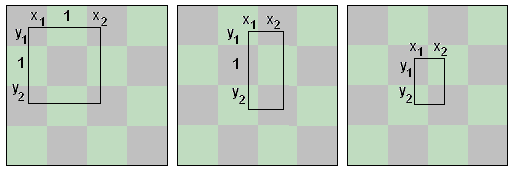# Rectangle on a Chessboard

A rectangle is drawn on a chessboard with sides parallel to the sides of the latter. A sufficiently small rectangle may be included in a single chessboard square, but, in general, a rectangle will contain regions of both chessboard colors. Is there a condition that guarantees that the areas of those regions are equal?

The problem can be recast in probabilistic terms: a given rectangle is placed randomly on a chessboard with sides parallel to the latter. What is the probability that it will contain the same amount of two colors?

### If you are reading this, your browser is not set to run Java applets. Try IE11 or Safari and declare the site https://www.cut-the-knot.org as trusted in the Java setup.What if applet does not run?A rectangle with sides parallel to the sides of a chessboard covers equal areas of the two colors if and only if one of the two conditions holds:

1. One of the sides of the rectangle is an even multiple of the side of a chessboard square. The dimension of the other side is irrelevant.

2. The center of the rectangle lies on a grid line.

(I am indebted to Nathan Bowler for pointing out an omission in the initial variant of the statement. Nathan continues the discussion elsewhere.)

For simplicity, let's call any segment double a square's side in length even.

The sufficiency (the "if" part) is pretty obvious. If, say, the vertical dimension of the rectangle is double a square's side, then in every vertical crossection of the rectangle segments of different colors add up to the same lengths. Also, if the center of the rectangle lies on a grid line, then by symmetry the statement is also true.

The applet shows that every rectangle can be split into at most three: one with an even horizontal dimension, one with an even vertical dimension, and one with both dimensions less than 2.

### If you are reading this, your browser is not set to run Java applets. Try IE11 or Safari and declare the site https://www.cut-the-knot.org as trusted in the Java setup.What if applet does not run?

The problem of showing the necessity, i.e. the "only if" part, is thus reduced to showing that in a rectangle with sides less than 2 there is more of one color than the other, provided the center of the rectangle does not lie on a grid line. There are three prototypical possibilities:In all cases,

0 < x1, x2 < 1 and 0 < y1, y2 < 1.

Also,

0 < x1 + x2 < 1

in the first case, and

0 < y1 + y2 < 1

in cases 1 and 2.

If A1 and A2 are total areas of the regions of the light and dark colors inside the rectangle, then, in the first case we get

A1 = x1 + x2 + y1 + y2.
A2 = (x1 + x2)(y1 + y2) + 1.

The difference of the two is

A1 - A2 = -(x1 + x2 - 1)(y1 + y2 - 1),

which is never 0.

In the second case we get

A1 = x1y1 + x2 + x1y2 = x1(y1 + y2) + x2.
A2 = x2y1 + x1 + x2y2 = x2(y1 + y2) + x1.

The difference of the two is

A1 - A2 = (x1 - x2)(y1 + y2 - 1),

which may be 0 iff x1 = x2, i.e, in the excluded case where the center of the rectangle lies on a grid line. The last case is treated similarly.

Due to the fact that the probability of a random rectangle having the center on a grid line is zero, the probabilistic recast has an unexpected solution: for a rectangle with an even side, the probability is 1; for the less fortunate rectangles, the probability is 0.The above proposition serves as a basis for a proof (by R. Rochberg and S. K. Stein) of a curious theorem of a relatively recent origin.

### Theorem

Whenever a rectangle is tiled by rectangles with parallel sides each of which has at least one integer side, then the ambient rectangle has at least one integer side.

### Proof

This is the proof #3 of the fourteen listed by S. Wagon in 1987.

Think of the rectangle as placed on a chessboard with the small square side of length 1/2 unit. The chessboard can be looked at as a 1/2×1/2 grid. Assume the lower left corner is at a node of this grid. By the above proposition, each of the tiles has an equal amount of two colors. Therefore, the tiled rectangle also has an equal amount of two colors. The proposition then implies the theorem: a rectangle covering equal areas of two colors is bound to have at least one even side, i.e. a side whose length is a multiple of twice the small square's side (1/2). The argument is curious: for a rectangle with a corner at a grid point, the two conditions above are equivalent. But, as we know, at least one of the two holds. Thus both do.

(A different proof that was found after Stan Wagon's article came out is discussed elsewhere. There is also a simple proof by math induction.)

### References

1. S. Wagon, Fourteen Proofs of a Result About Tiling a Rectangle, Am Math Monthly, Vol. 94, No. 7 (Aug. - Sept., 1987), 601-617.### Integer Rectangles### Geometric Probability